# DFA to Regular Expression | Arden’s Theorem

## DFA to Regular Expression-

The two popular methods for converting a given DFA to its regular expression are-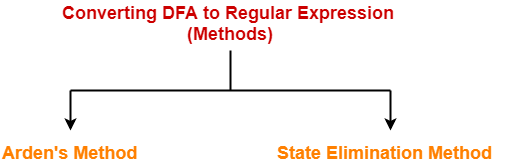1. Arden’s Method
2. State Elimination Method

## Arden’s Theorem-

Arden’s Theorem is popularly used to convert a given DFA to its regular expression.

It states that-

Let P and Q be two regular expressions over ∑.

If P does not contain a null string ∈, then-

R = Q + RP has a unique solution i.e. R = QP*

## Conditions-

To use Arden’s Theorem, following conditions must be satisfied-

• The transition diagram must not have any ∈ transitions.
• There must be only a single initial state.

## Steps-

To convert a given DFA to its regular expression using Arden’s Theorem, following steps are followed-

### Step-01:

• Form a equation for each state considering the transitions which comes towards that state.
• Add ‘∈’ in the equation of initial state.

### Step-02:

Bring final state in the form R = Q + RP to get the required regular expression.

## Important Notes-

### Note-01:

Arden’s Theorem can be used to find a regular expression for both DFA and NFA.

### Note-02:

If there exists multiple final states, then-

• Write a regular expression for each final state separately.
• Add all the regular expressions to get the final regular expression.

## Problem-01:

Find regular expression for the following DFA using Arden’s Theorem-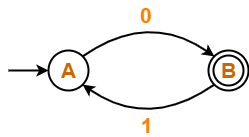## Solution-

### Step-01:

Form a equation for each state-

• A = ∈ + B.1 ……(1)
• B = A.0 ……(2)

### Step-02:

Bring final state in the form R = Q + RP.

Using (1) in (2), we get-

B = (∈ + B.1).0

B = ∈.0 + B.1.0

B = 0 + B.(1.0) ……(3)

Using Arden’s Theorem in (3), we get-

B = 0.(1.0)*

Thus, Regular Expression for the given DFA = 0(10)*

## Problem-02:

Find regular expression for the following DFA using Arden’s Theorem-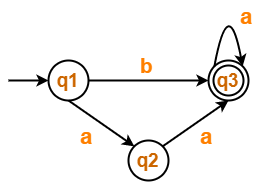## Solution-

### Step-01:

Form a equation for each state-

• q1 = ∈ ……(1)
• q2 = q1.a ……(2)
• q3 = q1.b + q2.a + q3.a …….(3)

### Step-02:

Bring final state in the form R = Q + RP.

Using (1) in (2), we get-

q2 = ∈.a

q2 = a …….(4)

Using (1) and (4) in (3), we get-

q3 = q1.b + q2.a + q3.a

q3 = ∈.b + a.a + q3.a

q3 = (b + a.a) + q3.a …….(5)

Using Arden’s Theorem in (5), we get-

q3 = (b + a.a)a*

Thus, Regular Expression for the given DFA = (b + aa)a*

## Problem-03:

Find regular expression for the following DFA using Arden’s Theorem-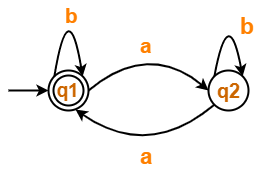## Solution-

### Step-01:

Form a equation for each state-

• q1 = ∈ + q1.b + q2.a ……(1)
• q2 = q1.a + q2.b ……(2)

### Step-02:

Bring final state in the form R = Q + RP.

Using Arden’s Theorem in (2), we get-

q2 = q1.a.b* …….(3)

Using (3) in (1), we get-

q1 = ∈ + q1.b + q1.a.b*.a

q1 = ∈ + q1.(b + a.b*.a) …….(4)

Using Arden’s Theorem in (4), we get-

q1 = ∈.(b + a.b*.a)*

q1 = (b + a.b*.a)*

Thus, Regular Expression for the given DFA = (b + a.b*.a)*

## Problem-04:

Find regular expression for the following DFA using Arden’s Theorem-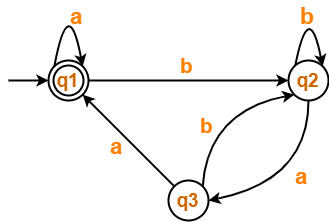## Solution-

### Step-01:

Form a equation for each state-

• q1 = ∈ + q1.a + q3.a ……(1)
• q2 = q1.b + q2.b + q3.b ……(2)
• q3 = q2.a …….(3)

### Step-02:

Bring final state in the form R = Q + RP.

Using (3) in (2), we get-

q2 = q1.b + q2.b + q2.a.b

q2 = q1.b + q2.(b + a.b) …….(4)

Using Arden’s Theorem in (4), we get-

q2 = q1.b.(b + a.b)* …….(5)

Using (5) in (3), we get-

q3 = q1.b.(b + a.b)*.a …….(6)

Using (6) in (1), we get-

q1 = ∈ + q1.a + q1.b.(b + a.b)*.a.a

q1 = ∈ + q1.(a + b.(b + a.b)*.a.a) …….(7)

Using Arden’s Theorem in (7), we get-

q1 = ∈.(a + b.(b + a.b)*.a.a)*

q1 = (a + b.(b + a.b)*.a.a)*

Thus, Regular Expression for the given DFA = (a + b(b + ab)*aa)*

To gain better understanding about Arden’s Theorem,

Watch this Video Lecture

Next Article- Non-Deterministic Finite Automata

Get more notes and other study material of Theory of Automata and Computation.

Watch video lectures by visiting our YouTube channel LearnVidFun.

SummaryArticle Name
DFA to Regular Expression | Arden's Theorem
Description
Arden's Theorem is a popular method to convert DFA to regular expression. Arden's Theorem Examples. Problems on converting DFA to Regular Expression Using Arden's Theorem.
Author
Publisher Name
Gate Vidyalay
Publisher Logo